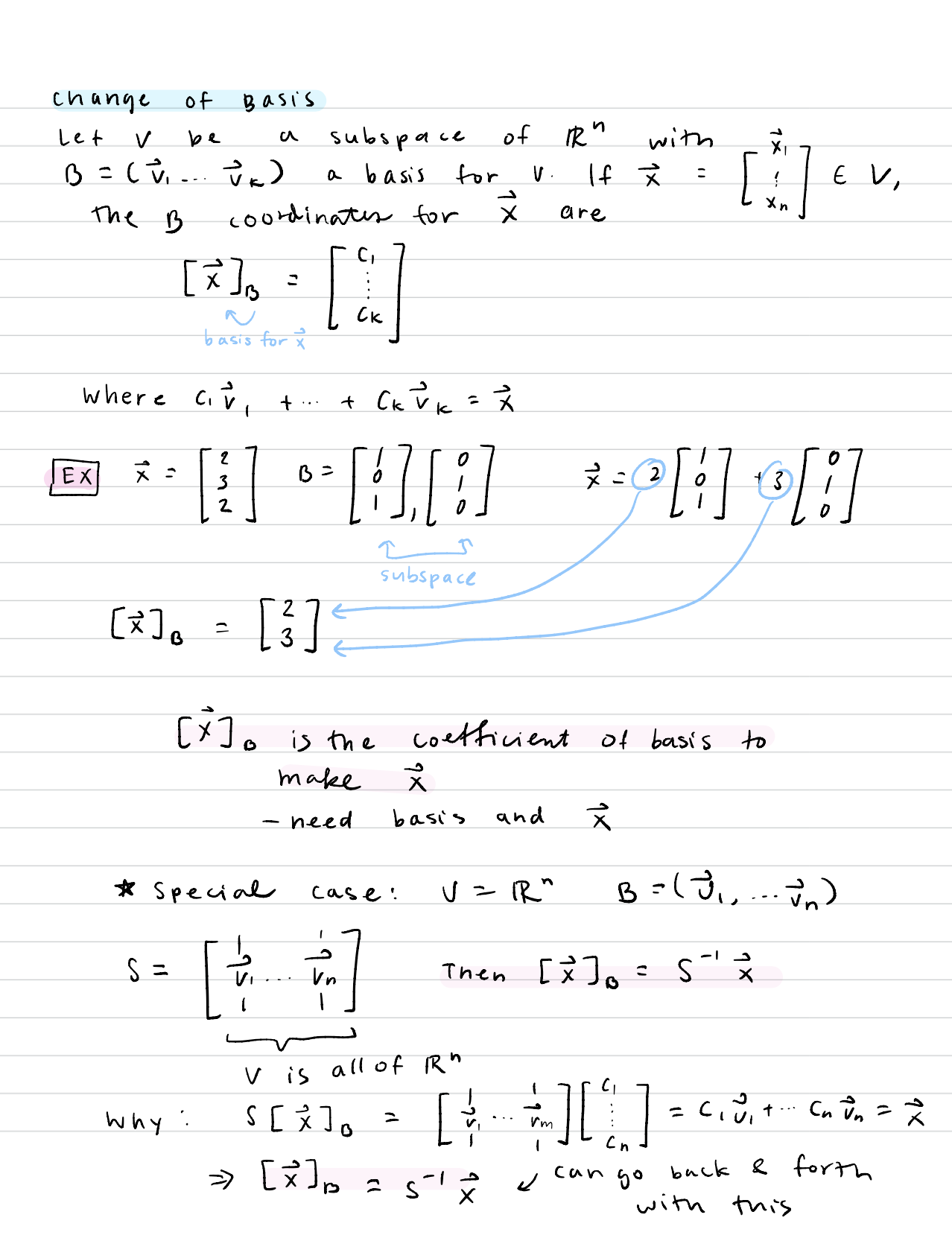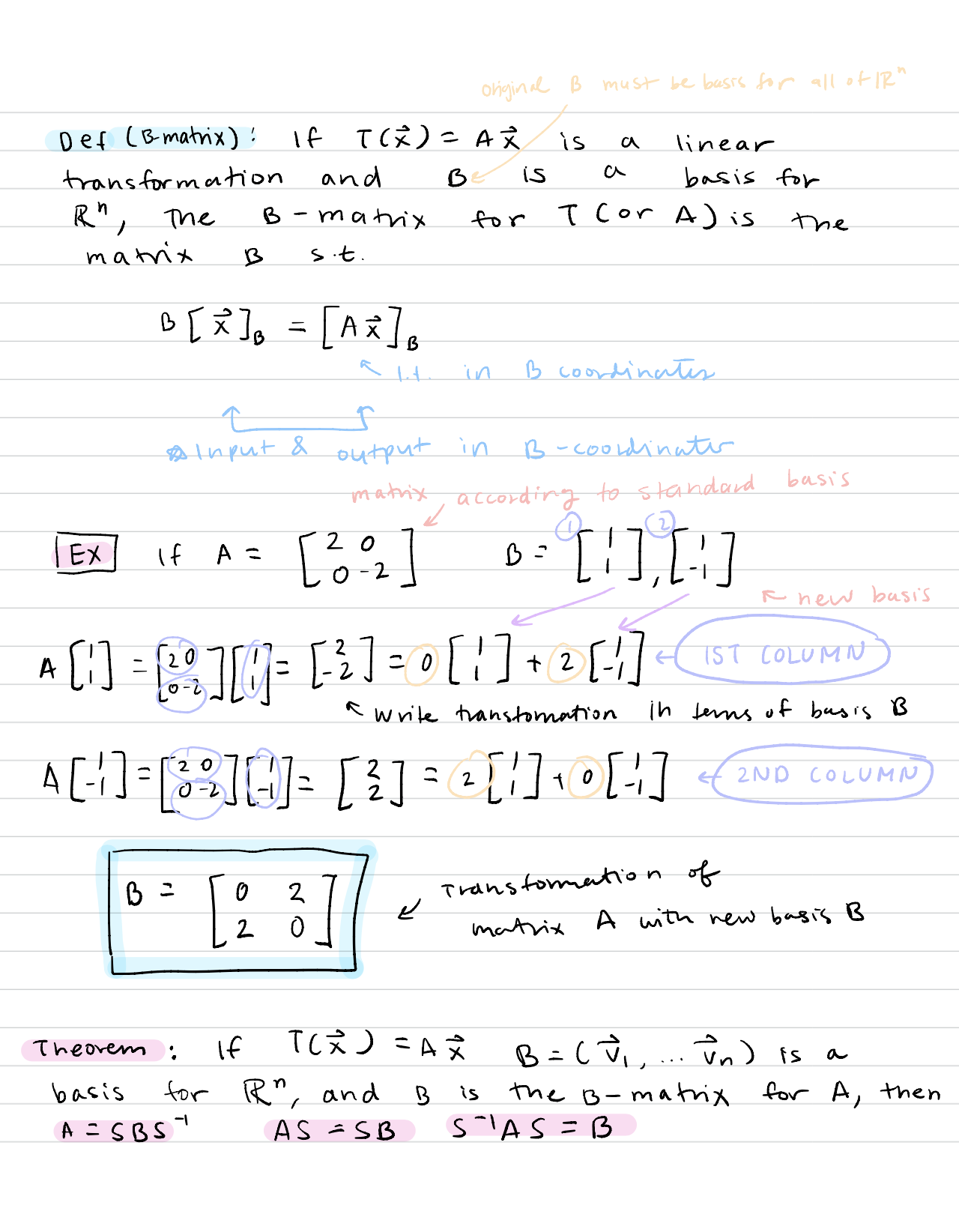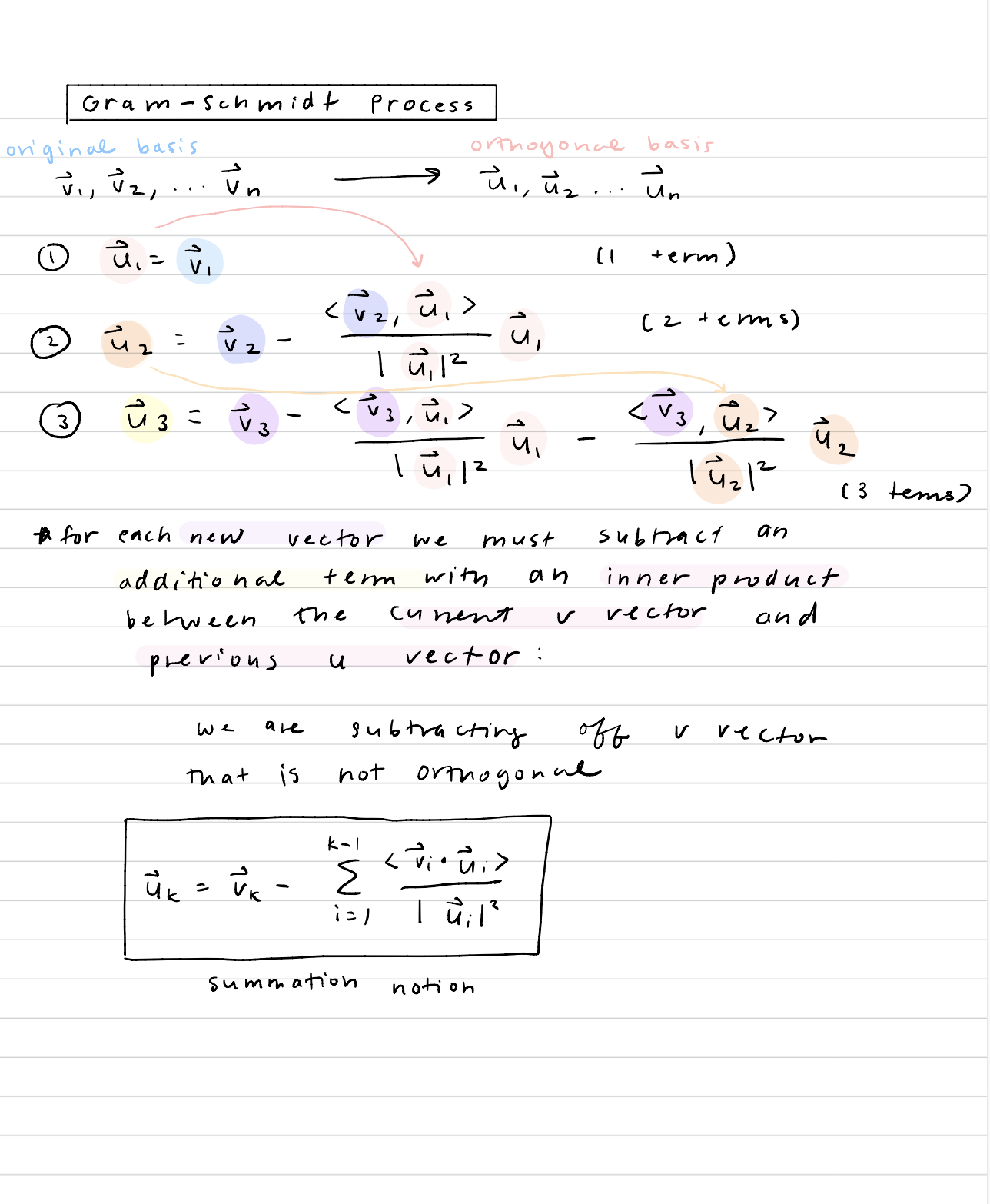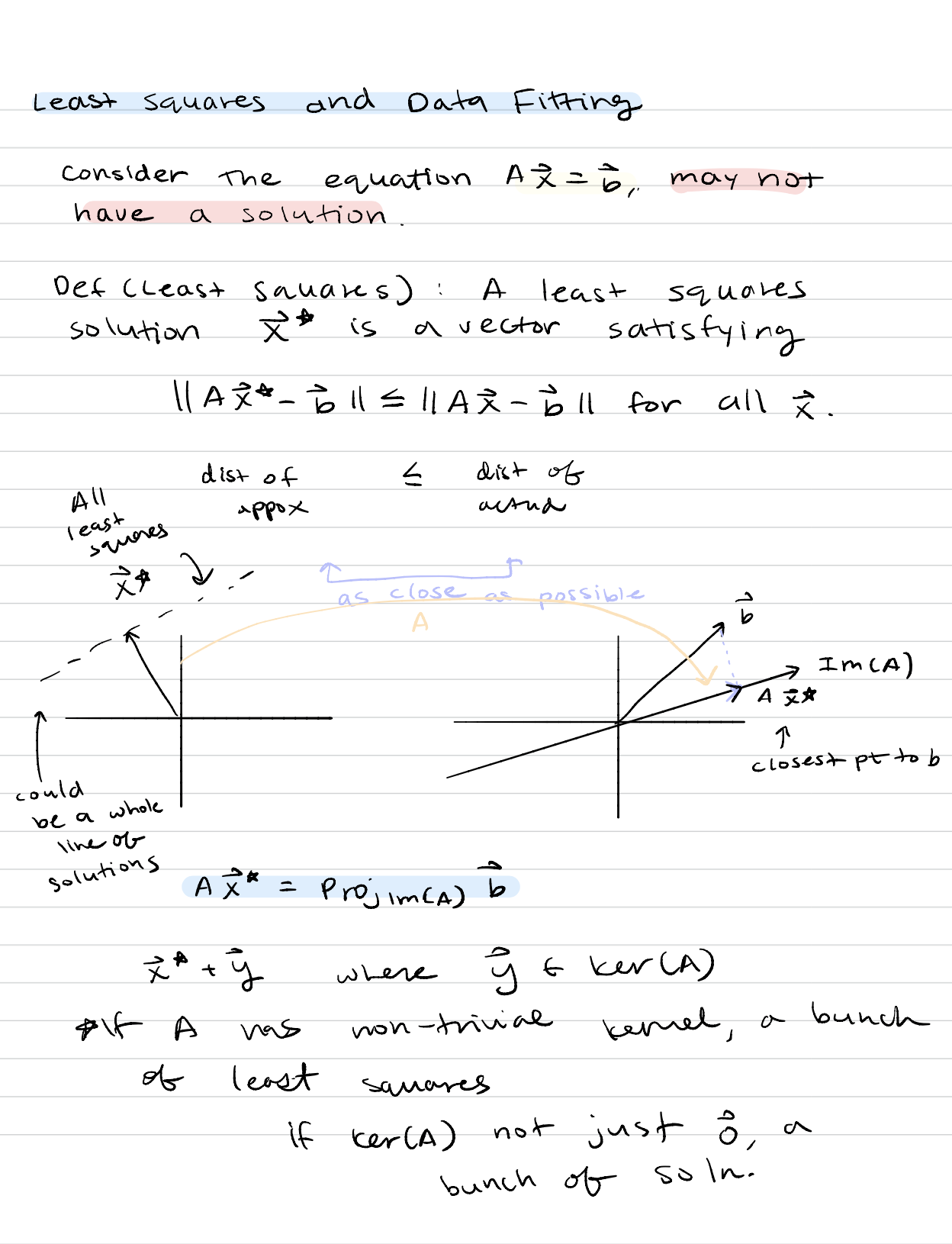# Linear Algebra Concepts

Since I’m studying for my Linear Algebra Midterm 2, I would like to share some cool concepts or algorithms we learned in class. My notes aren’t as neat as they are usually, but here are some cool things:

1. Change of Basis – essentially writing a matrix with  basis for R^n into a matrix with another basis (so converting the matrix into another basis “terms” or “language”)

This is a really great video explanation describing the concept of it: Change of Basis

Here are my notes from it. Here in my notes, [x]B is the new vector in terms of the new basis:2. Gram-Schmidt Process – a way to solve for the orthogonal and orthonormal basis from an original basis. I thought the algorithm and pattern behind it was interesting since, for each new vector, you essentially subtract the “not orthogonal part” to force the remainder to be orthogonal only3. Least Squares and Data Fitting – least squares is basically when there is no actual perfect solution to a linear transformation or matrix solution, but you try to find the one that is closest to it. I thought that was pretty deep on both a mathematical and philosophical level because it’s saying that although one cannot reach perfection, they must strive towards it. Sort of like my Model United Nations essay for college applications. If you have a lot of points on a 2D graph, the least squares regression would be the line that best fits the points on a line. I thought this as choosing someone/raising someone too–you can’t get make the perfect person and someone won’t have all the qualities you want, but you can get close enough.

Anyways, here are my notes form it:## 3 Replies to “Linear Algebra Concepts”

1.Lia Hagadone says:

This actually answered my drawback, thanks!

2.agario private server says:

ally, but here are some cool things:

Change of Basis – essentially writing a matrix with basis for R^n into a matrix with another basis (so converting the matrix into another basis “terms” or “language”)

3.cast ajans says:

thanks for sharing